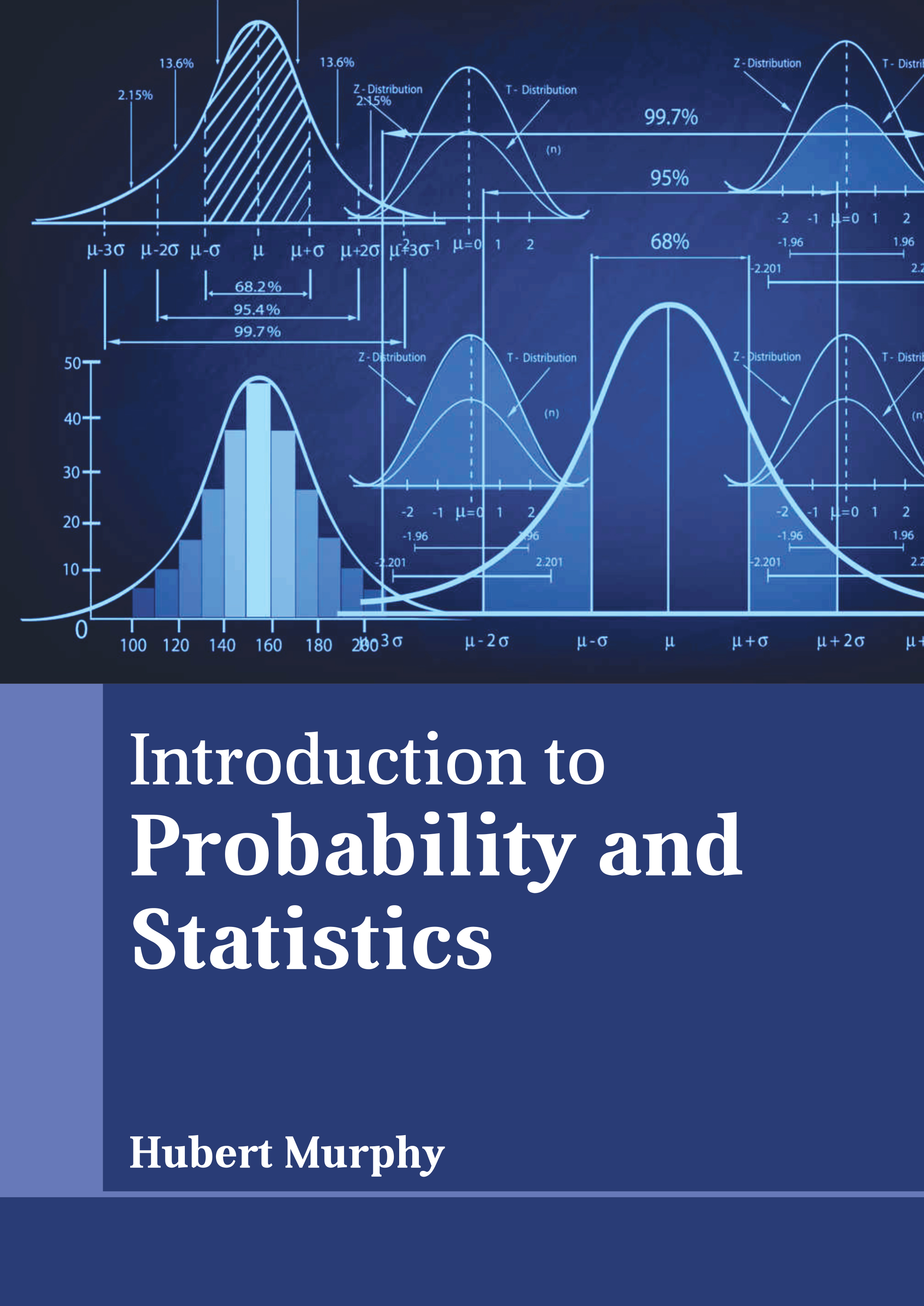# Books /Introduction to Probability and Statistics

BROWSE BY

### Introduction to Probability and Statistics9781639897155

Author

Hubert Murphy

English

##### Publication Year

2023

Category :

Mathematics and Statistics - Probability and Statistics

\$ 170.95

##### Description

Probability and statistics are two sub-disciplines of mathematics. Probability is related to the chance of occurrence of an event, whereas statistics is involved in handling data utilizing various techniques. Probability deals with numerical explanations of possible occurrence of an event or likelihood of a proposition being true. The probability of an event is a number between 0 and 1, wherein 0 represents impossibility and 1 represents certainty. The higher the probability, it is more likely that the particular event will happen. Statistics deals with gathering, organizing, interpreting, analyzing, and presenting data. It is a common practice to start with a statistical model or statistical population to be researched when applying statistics to a scientific, social, or industrial problem. This book provides comprehensive insights into the fields of probability and statistics. It presents this complex subject in the most comprehensible and easy to understand language. This book will provide comprehensive knowledge to the readers.